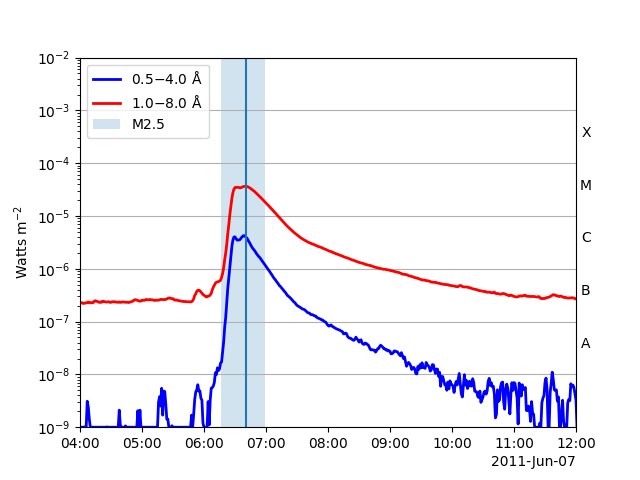# Flare times on a GOES XRS plot¶

How to plot flare times as provided by the HEK on a GOES XRS plot.

```import matplotlib.pyplot as plt

from sunpy.net import Fido
from sunpy.net import attrs as a
from sunpy.time import parse_time
from sunpy.timeseries import TimeSeries
```

Let’s grab GOES XRS data for a particular time of interest and the HEK flare data for this time from the NOAA Space Weather Prediction Center (SWPC).

```tr = a.Time('2011-06-07 04:00', '2011-06-07 12:00')
results = Fido.search(tr, a.Instrument.xrs & a.goes.SatelliteNumber(15) | a.hek.FL & (a.hek.FRM.Name == 'SWPC'))  # NOQA
```

```files = Fido.fetch(results)
goes = TimeSeries(files)
```

Next let’s retrieve `HEKTable` from the Fido result and then load the first row from HEK results into `flares_hek`.

```hek_results = results['hek']
flares_hek = hek_results
```

Lets plot everything together.

```plt.figure()
goes.plot()
plt.axvline(parse_time(flares_hek['event_peaktime']).datetime)
plt.axvspan(parse_time(flares_hek['event_starttime']).datetime,
parse_time(flares_hek['event_endtime']).datetime,
alpha=0.2, label=flares_hek['fl_goescls'])
plt.legend(loc=2)
plt.yscale('log')

plt.show()
```Total running time of the script: ( 0 minutes 6.121 seconds)

Gallery generated by Sphinx-Gallery